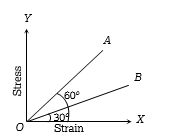If the force constant of a wire is K, the work done in increasing the length of the wire by l is

(a) $Kl/2$                                   (b) $Kl$

(c) $K{l}^{2}/2$                                 (d) $K{l}^{2}$

Concept Questions :-

Stress-strain
High Yielding Test Series + Question Bank - NEET 2020

Difficulty Level:

When strain is produced in a body within elastic limit, its internal energy

(a) Remains constant                   (b) Decreases

(c) Increases                               (d) None of the above

Concept Questions :-

Elasticity
High Yielding Test Series + Question Bank - NEET 2020

Difficulty Level:

When shearing force is applied on a body, then the elastic potential energy is stored in it. On removing the force, this energy

1. Converts into kinetic energy

2. Converts into heat energy

3. Remains as potential energy

4. None of the above

Concept Questions :-

Elasticity

Difficulty Level:

A wire is suspended by one end. At the other end a weight equivalent to 20 N force is applied. If the increase in length is 1.0 mm, the increase in energy of the wire will be

(a) 0.01 J                                (b) 0.02 J

(c) 0.04 J                                (d) 1.00 J

Concept Questions :-

Stress-strain
High Yielding Test Series + Question Bank - NEET 2020

Difficulty Level:

The ratio of Young's modulus of the material of two wires is 2 : 3. If the same stress is applied on both, then the ratio of elastic energy per unit volume will be-

(a) 3 : 2                                   (b) 2 : 3

(c) 3 : 4                                    (d) 4 : 3

Concept Questions :-

Stress-strain
High Yielding Test Series + Question Bank - NEET 2020

Difficulty Level:

The stress versus strain graphs for wires of two materials A and B are as shown in the figure. If ${Y}_{A}$ and ${Y}_{B}$ are the Young ‘s modulii of the materials, then(a) ${Y}_{B}=2{Y}_{A}$

(b) ${Y}_{A}={Y}_{B}$

(c) ${Y}_{B}=3{Y}_{A}$

(d) ${Y}_{A}=3{Y}_{B}$

Concept Questions :-

Stress-strain curve
High Yielding Test Series + Question Bank - NEET 2020

Difficulty Level:

The Young's modulus of a wire is Y.  If the energy per unit volume is E, then the strain will be

(a) $\sqrt{\frac{2E}{Y}}$                                         (b) $\sqrt{2EY}$

(c) $EY$                                              (d) $\frac{E}{Y}$

Concept Questions :-

Stress-strain
High Yielding Test Series + Question Bank - NEET 2020

Difficulty Level:

If a spring extends by x on loading, then the energy stored by the spring is (if T is tension in the spring and k is spring constant)

(a) $\frac{{T}^{2}}{2x}$                                      (b) $\frac{{T}^{2}}{2k}$

(c) $\frac{2x}{{T}^{2}}$                                      (d) $\frac{2{T}^{2}}{k}$

Concept Questions :-

Stress-strain
High Yielding Test Series + Question Bank - NEET 2020

Difficulty Level:

When a force is applied on a wire of uniform cross-sectional area $3×{10}^{-6}{m}^{2}$ and length 4m, the increase in length is 1 mm. Energy stored in it will be $\left(Y=2×{10}^{11}N/{m}^{2}\right)$

1. 6250 J                               2. 0.177 J

3. 0.075 J                              4. 0.150 J

Concept Questions :-

Stress-strain
High Yielding Test Series + Question Bank - NEET 2020

Difficulty Level:

A wire of length L and cross-sectional area A is made of a material of Young's modulus Y. It is stretched by an amount x. The work done is -

(a) $\frac{YxA}{2L}$                             (b) $\frac{Y{x}^{2}A}{L}$

(c) $\frac{Y{x}^{2}A}{2L}$                           (d) $\frac{2Y{x}^{2}A}{L}$

Concept Questions :-

Hooke's law